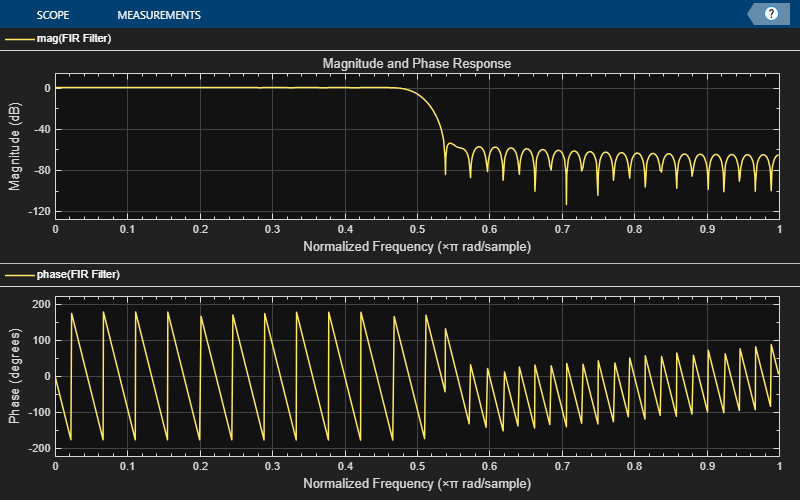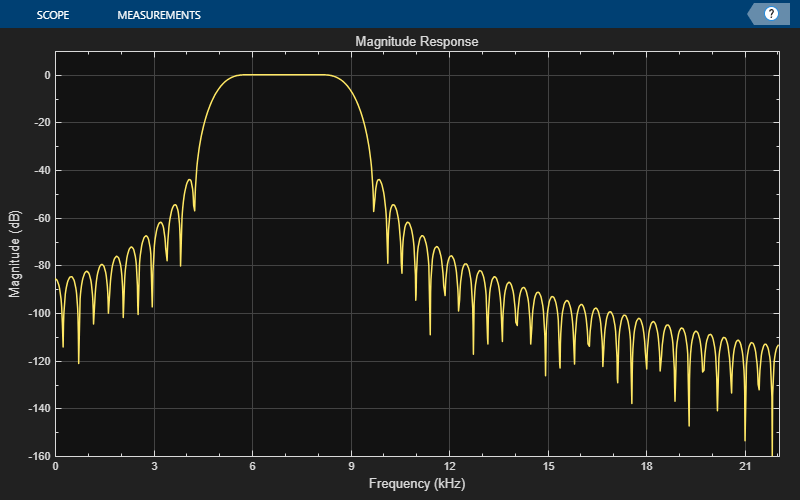# dsp.DynamicFilterVisualizer

Display time-varying magnitude response of digital filters

## Description

The `dsp.DynamicFilterVisualizer` object displays the magnitude response of time-varying digital filters or time-varying filter coefficients. The input to this object can be a filter coefficients vector or a filter System object™.

Using the dynamic filter visualizer, you can configure the plot settings, measure the signal statistics, find the peak values, place the data cursors, and so on from the interface of the visualizer. For details, see Configure Array Plot.

## Creation

### Syntax

``dfv = dsp.DynamicFilterVisualizer``
``dfv = dsp.DynamicFilterVisualizer(nfft)``
``dfv = dsp.DynamicFilterVisualizer(nfft,Fs)``
``dfv = dsp.DynamicFilterVisualizer(nfft,Fs,range)``
``dfv = dsp.DynamicFilterVisualizer(Name,Value)``

### Description

example

````dfv = dsp.DynamicFilterVisualizer` returns a dynamic filter visualizer object, `dfv`, that displays the magnitude response of digital filters or filter coefficients.```
````dfv = dsp.DynamicFilterVisualizer(nfft)` returns a dynamic filter visualizer with the `FFTLength` property set to `nfft`.```
````dfv = dsp.DynamicFilterVisualizer(nfft,Fs)` returns a dynamic filter visualizer with the `FFTLength` property set to `nfft` and the `SampleRate` property set to `Fs`.```
````dfv = dsp.DynamicFilterVisualizer(nfft,Fs,range)` returns a dynamic filter visualizer with the `FFTLength` property set to `nfft`, the `SampleRate` property set to `Fs`, and the `FrequencyRange` property set to `range`.```

example

````dfv = dsp.DynamicFilterVisualizer(Name,Value)` returns a dynamic filter visualizer with each specified property set to the specified value. You can specify name-value pair arguments in any order.```

## Properties

expand all

FFT length that the dynamic filter visualizer uses to compute spectral estimates, specified as a positive integer.

Data Types: `single` | `double` | `int8` | `int16` | `int32` | `int64` | `uint8` | `uint16` | `uint32` | `uint64`

Sampling rate of the input signal, specified as a real positive scalar in Hz.

Data Types: `single` | `double` | `int8` | `int16` | `int32` | `int64` | `uint8` | `uint16` | `uint32` | `uint64`

Range of the frequency axis, specified as a two-element numeric vector that is monotonically increasing and of the form [fmin, fmax]. The upper limit must be less than or equal to Fs/2, where Fs is the value specified in `SampleRate`.

Tunable: Yes

Data Types: `single` | `double` | `int8` | `int16` | `int32` | `int64` | `uint8` | `uint16` | `uint32` | `uint64`

X-axis scale, specified as either `'Linear'` or `'Log'`.

Tunable: Yes

Y-axis units, specified as one of the following:

• `'Magnitude'`

• `'Magnitude (dB)'`

• `'Magnitude squared'`

Tunable: Yes

## Visualization

Caption to display on the Dynamic Filter Visualizer window, specified as a character vector or a string scalar.

Example: `'Dynamic Filter Visualizer'`

Example: `"Dynamic Filter Visualizer"`

Tunable: Yes

Display title, specified as a character vector or a string scalar.

Example: `'Magnitude Response'`

Example: `"Magnitude Response"`

Tunable: Yes

Y-axis limits, specified as a two-element numeric vector with the second element greater than the first element and of the form [ymin, ymax].

Tunable: Yes

Data Types: `single` | `double` | `int8` | `int16` | `int32` | `int64` | `uint8` | `uint16` | `uint32` | `uint64`

When this property is set to `false`, no legend is displayed. When this property is set to `true`, a legend with automatic string labels for each input filter is displayed.

Tunable: Yes

Data Types: `logical`

Set this property to a cell array of character vectors to label the input filters in the legend. The default is an empty cell array. When this property is set to an empty cell array, the filters are named by default names, such as `Filter 1`, `Filter 2`, and so on.

Tunable: Yes

Upper limit spectral mask, specified as a two-column matrix. The first column represents the frequency values (Hz), and the second column represents the magnitude spectrum of the upper limit mask.

Data Types: `single` | `double` | `int8` | `int16` | `int32` | `int64` | `uint8` | `uint16` | `uint32` | `uint64`

Lower limit spectral mask, specified as a two-column matrix. The first column represents the frequency values (Hz), and the second column represents the magnitude spectrum of the lower limit mask.

Data Types: `single` | `double` | `int8` | `int16` | `int32` | `int64` | `uint8` | `uint16` | `uint32` | `uint64`

Scope window position in pixels, specified as a four-element double vector of the form [left bottom width height]. The default value of this property is dependent on the screen resolution, and is such that the window is positioned in the center of the screen, with a width and height of `410` and `300` pixels, respectively.

Tunable: Yes

Data Types: `single` | `double` | `int8` | `int16` | `int32` | `int64` | `uint8` | `uint16` | `uint32` | `uint64`

## Usage

### Syntax

``dfv(filt)``
``dfv(B,A)``

### Description

````dfv(filt)` displays the time-varying magnitude response of the object filter, `filt`, in the Dynamic Filter Visualizer figure, as long as `filt` has a valid `freqz()` implementation.```
````dfv(B,A)` displays the magnitude response for the digital filters with numerator and denominator polynomial coefficients stored in `B1` and `A1`, `B2` and `A2`, ..., and `BN` and `AN`, respectively.```

### Input Arguments

expand all

Input filter System object with a valid `freqz()` implementation.

Numerator polynomial coefficients, specified as a row vector.

Data Types: `single` | `double`

Denominator polynomial coefficients, specified as a:

• scalar –– The filter is an FIR filter.

• row vector –– The filter is an IIR filter.

Data Types: `single` | `double`

## Object Functions

expand all

 `step` Display time-varying magnitude response
 `show` Display scope window `hide` Hide scope window

## Examples

collapse all

Design an FIR filter with time-varying magnitude response. Plot this varying response on a dynamic filter visualizer.

Create a `dsp.DynamicFilterVisualizer` object.

`dfv = dsp.DynamicFilterVisualizer('YLimits',[-120 10])`
```dfv = DynamicFilterVisualizer with properties: FFTLength: 2048 SampleRate: 44100 FrequencyRange: [0 22050] XScale: 'Linear' MagnitudeDisplay: 'Magnitude (dB)' Visualization Name: 'Dynamic Filter Visualizer' Title: 'Magnitude Response' YLimits: [-120 10] ShowLegend: 0 FilterNames: {''} UpperMask: Inf LowerMask: -Inf Position: [240 262 800 500] ```

Vary the cutoff frequency of the FIR filter, k, from `0.1` to `0.5` in increments of `0.001`. View the varying magnitude response using the dynamic filter visualizer.

```for k = 0.1:0.001:0.5 b = fir1(90,k); dfv(b,1); end```Visualize the varying magnitude response of the variable bandwidth FIR filter using the dyamic filter visualizer.

Create a `dsp.DynamicFilterVisualizer` object.

`dfv = dsp.DynamicFilterVisualizer('YLimits',[-160 10])`
```dfv = DynamicFilterVisualizer with properties: FFTLength: 2048 SampleRate: 44100 FrequencyRange: [0 22050] XScale: 'Linear' MagnitudeDisplay: 'Magnitude (dB)' Visualization Name: 'Dynamic Filter Visualizer' Title: 'Magnitude Response' YLimits: [-160 10] ShowLegend: 0 FilterNames: {''} UpperMask: Inf LowerMask: -Inf Position: [240 262 800 500] ```

Design a bandpass variable bandwidth FIR filter with a center frequency of 5 kHz and a bandwidth of 4 kHz.

```Fs = 44100; vbw = dsp.VariableBandwidthFIRFilter('FilterType','Bandpass',... 'FilterOrder',100,... 'SampleRate',Fs,... 'CenterFrequency',5e3,... 'Bandwidth',4e3);```

Vary the center frequency of the filter. Visualize the varying magnitude response of the filter using the `dsp.DynamicFilterVisualizer` object.

```for idx = 1:100 dfv(vbw); vbw.CenterFrequency = vbw.CenterFrequency + 20; end```## Version History

Introduced in R2018b